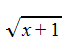# The Export Process

Any export of expressions results in a string of text. This topic describes the process of getting from the original expression to this string.

When an expression is exported, an export function is called. Which exporters are defined and the name of the function that will be called for each is determined by the GetExporters function in the Code namespace.

Typically, a document will refer to a library that contains the export function. For example, a document with have a reference to the LaTeX library if export to LaTeX is needed.

Any valid export function will return an expression of type Text. Most commonly, the function will return a TextList object containing a list of objects, each of which is of type Text. These objects are converted directly to text by the Sym application.

The conversion to text is straight forward. Most commonly, the output of the export function is a TextList object that contains a list of Text objects. The Text objects are just replaced with the text in the object during the conversion process. Any object other than the Text object is just converted to a text string by first converting each of the parameters to text, then combining the name of the object followed by an opening parentheses, the text for each parameter (separated by commas) and a closing parentheses.The output of the LaTeX export function is actually five Text objects in a TextList. The five Text objects contain the strings:

"\sqrt{"

"x"

"+"

"1"

"}"

The export process just combines this into a single string

"\sqrt{x+1}"

As a second example, suppose that you create the expression:and that the LaTeX exporter doesn't have a specific clause for the "fn" object. In this case, the TextList contains:

"fn"

"\left("

(the same five text objects as in the first example)

"\right)"

giving a resulting string of "fn\left(\sqrt{x+1}\right)". The LaTeX exporter also wraps the expression in text to indicate that the expression is a equation, so the complete export of our example is the text:

\begin{equation*}

fn\left(\sqrt{x+1}\right)

\end{equation*}